# Stochastic differential

A random interval function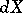defined by the formulafor every processin the class of semi-martingales, with respect to a stochastic basis. In the family of stochastic differentials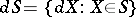one introduces addition, multiplication by a process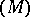and the product operationaccording to the following formulas:;(a stochastic integral,being a locally bounded predictable process which is adapted to the filtration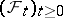);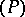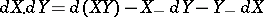, whereand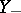are the left-continuous versions ofand.

It then turns out thatwhereis an arbitrary decomposition of the interval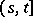, l.i.p. is the limit in probability, and.

In stochastic analysis, the principle of "differentiation" of random functions, or Itô formula, is of importance: If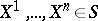and the function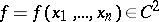, then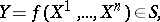and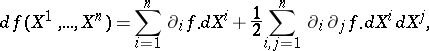(1)

where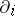is the partial derivative with respect to the-th coordinate. In particular, it can be inferred from (1) that if, then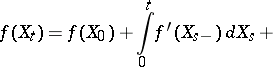(2)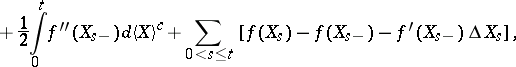where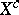is the continuous martingale part of,.

Formula (2) can be given the following form: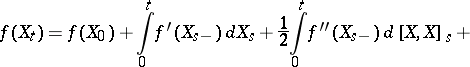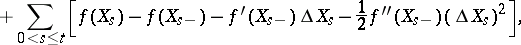where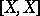is the quadratic variation of.